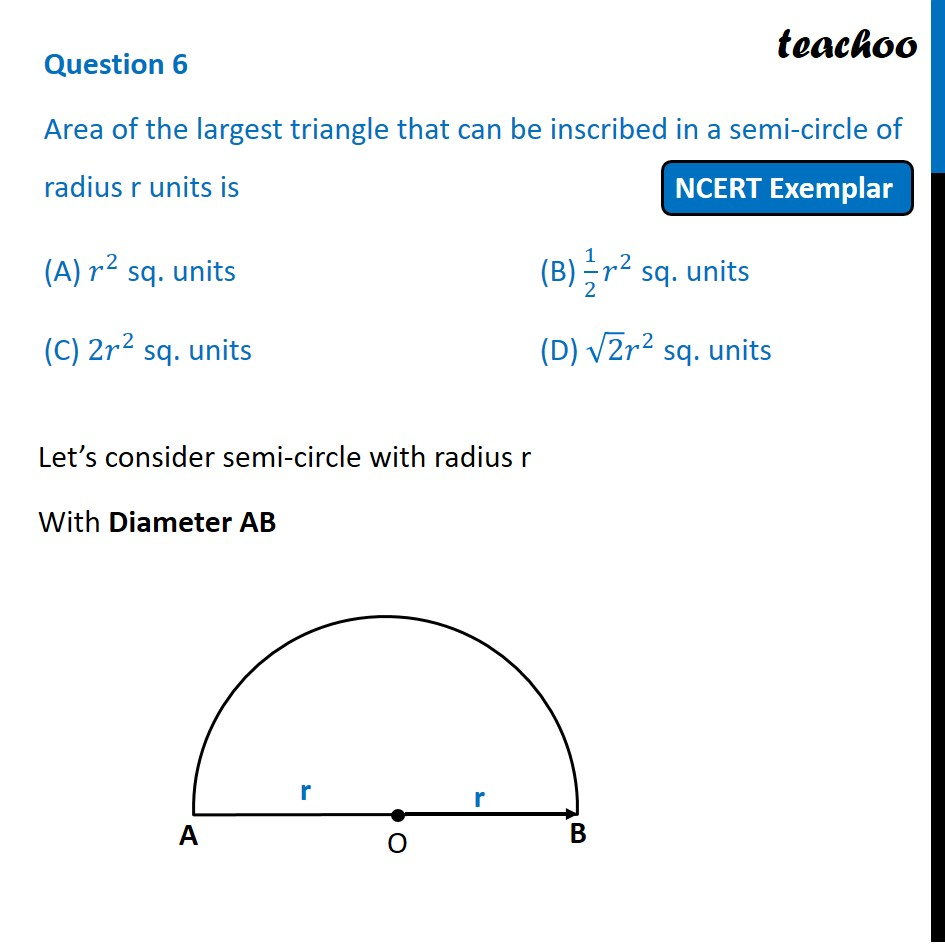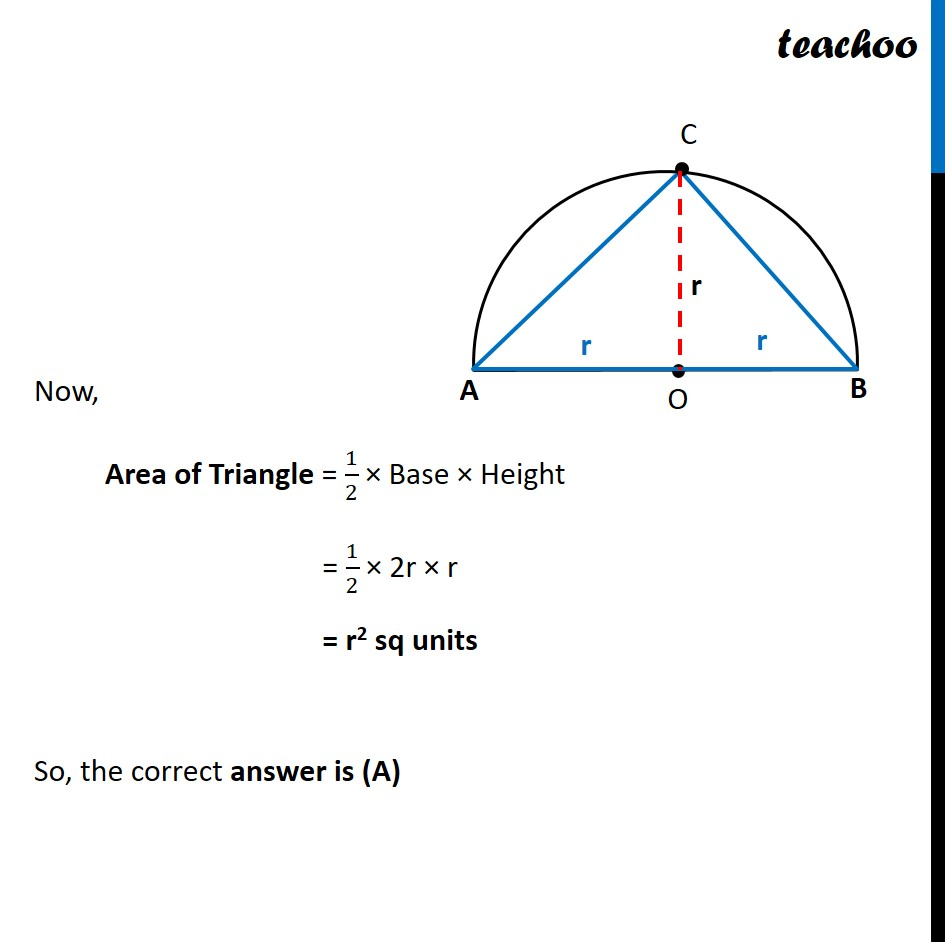NCERT Exemplar - MCQ

Chapter 11 Class 10 Areas related to Circles
Serial order wise

## (C) 2r 2   sq. units   (D) √2 r 2 sq. unitsLearn in your speed, with individual attention - Teachoo Maths 1-on-1 Class

### Transcript

Question 6 Area of the largest triangle that can be inscribed in a semi-circle of radius r units is (A) 𝑟^2 sq. units (B) 1/2 𝑟^2 sq. units (C) 2𝑟^2 sq. units (D) √2 𝑟^2 sq. units Let’s consider semi-circle with radius r With Diameter AB Now, Area of Triangle = 1/2 × Base × Height = 1/2 × 2r × r = r2 sq units So, the correct answer is (A)